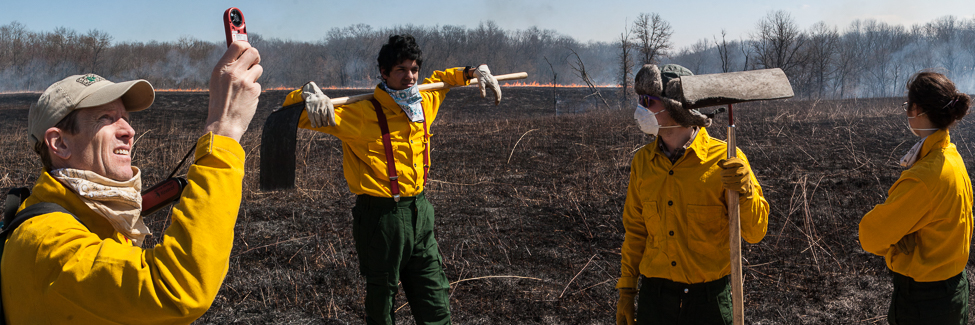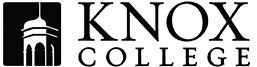Search
Knox Together: COVID-19 Plans & Policies Visitor Policy Give Now# Courses

Stuart Allison

Chair of Mathematics & Watson Bartlett Professor of Biology

Galesburg, IL 61401

309-341-7185

sallison@​knox.eduMATH 121 Mathematical Ideas

An introduction to the history and concepts of elementary mathematics. Topics may include: properties of number systems, geometry, analytic geometry, mathematical modeling, and probability and statistics. Designed for non-majors. QR; Offered every year, usually WI and SP; Staff

MATH 123 Math for Quantitative Disciplines

A foundational course for students pursuing further studies in fields which employ statistics, applied calculus, or discrete mathematics. The course will cover the elements of statistics and probability, functions and equation solving, finance, optimization, and logic in an applied context. QR; Offered every year, usually FA and SP; Staff

MATH 131 Functions

An introduction to the concept of a function and its graph. Polynomial and rational functions, logarithmic and exponential functions, and trigonometric functions. Examination of the relationship between algebraic and graphical formulations of ideas and concepts. Prerequisite(s): 3 years college preparatory mathematics or permission of the instructor; QR; Credit cannot be earned for both MATH 131 and CTL 130; Staff

MATH 145 Applied Calculus

A brief survey of differential and integral calculus from an applied perspective, including some material from multivariate calculus. Mathematical modeling with functions, derivatives, optimization, integration, elementary differential equations, partial derivatives. Prerequisite(s): Appropriate math placement level or MATH 123; QR; Offered every year, usually FA and WI; Staff

MATH 146 Applied Calculus II

Topics include a brief review of differential calculus and the fundamental theorem, applications of and techniques for evaluating the integral, including elementary multivariate integrals. Also includes Taylor series and further discussion of differential equations. The course is taught laboratory-style. Prerequisite(s): MATH 145, MATH 151, or equivalent; QR; Staff

MATH 151 Calculus I

An introduction to the theory and applications of the differential calculus. Limits, continuity, differentiation, approximation, and optimization. Prerequisite(s): MATH 131 or three years of college preparatory mathematics, including trigonometry, and appropriate placement level; QR; Offered every year, FA and WI; Staff

MATH 152 Calculus II

A continuation of MATH 151. An introduction to the theory and applications of the integral calculus as well as an introduction to infinite series and parametric equations. Prerequisite(s): MATH 151; QR; Offered every year, WI and SP; Staff

MATH 175 Discrete Mathematics

A study of discrete mathematical structures. Logic and proof, set theory, relations and functions, ideas of order and equivalence, and graphs. Prerequisite(s): MATH 123 and CS 141, or MATH 145 (or higher); QR; Offered every year SP; Staff

MATH 185 Introduction to Linear Algebra

An introduction to the fundamental properties of matrices, vector spaces, and linear transformations, with applications to a variety of disciplines. Course topics include systems of linear equations, matrix arithmetic, spanning, independence, bases, dimension, inner products, orthogonality, projections, eigenvalues and eigenvectors, and diagonalization. Applications may include least squares estimation, discrete dynamical systems, economic input-output models, and linear programming. Prerequisite(s): Math 145 or higher, or permission of the instructor; QR; Offered every year; Staff

MATH 205 Calculus III

An introduction to the calculus of functions of several variables and vector-valued functions. Limits, continuity, differentiation, and multiple integration. Prerequisite(s): MATH 152 or permission of the instructor; QR; Offered every year, FA and SP; Staff

MATH 210 Linear Algebra I

A study of the fundamental properties and applications of finite dimensional vector spaces, linear transformations, and matrices. Spanning, independence, bases, inner products, orthogonality, eigenvalues and eigenvectors, diagonalization. Prerequisite(s): MATH 152 and MATH 185 or permission of the instructor; Offered every year; Staff

MATH 215 Vector Calculus

A study of vector fields and the calculus of vector differential operators (gradient, divergence, curl, Laplacian), potential functions and conservative fields, line and surface integrals, the theorems of Green, Gauss, and Stokes. Applications. Prerequisite(s): MATH 205; Usually offered in alternate years; Staff

MATH 216 Foundations of Geometry

A study of the axiomatic structure and historical development of two-dimensional geometry, with an emphasis on proofs. Incidence geometry, geometry of flat and curved spaces, projective geometry, and Euclidean models for hyperbolic geometry. Historical implications of the existence of non-Euclidean geometries. Prerequisite(s): MATH 152; A. Leahy

MATH 217 Number Theory

A study of the properties of the natural numbers. Prime numbers, divisibility, congruences, Diophantine equations, and applications to cryptography. Prerequisite(s): MATH 152; Offered in alternate years, usually FA; M. Armon

MATH 218 History of Mathematics

A study of the evolution of mathematical ideas from ancient to modern times. Prerequisite(s): MATH 152; A. Leahy

MATH 225 Linear Models and Statistical Software

This course develops further the ideas and techniques that were introduced in STAT 200 relative to regression modeling and experimental design, understood as instances of a matrix linear model. In addition, the student becomes familiar with at least one leading statistical package for performing the intensive calculations necessary to analyze data. Topics include linear, non-linear, and multiple regression, model-building with both quantitative and qualitative variables, model-checking, logistic regression, experimental design principles, ANOVA for one-, two-, and multiple factor experiments, and multiple comparisons. Prerequisite(s): STAT 200, MATH 145 or 151, and MATH 185; Cross Listing: STAT 225; Offered every year; O. Forsberg

MATH 227 Introductory Financial Mathematics

An introduction to the mathematics of finance including interest, present value, annuities, probability modeling for finance, portfolio optimization, utility theory, and valuation of bonds, futures and options. Prerequisite(s): MATH 152 or permission of the instructor; Offered in alternate years, usually FA; K. Hastings

MATH 230 Differential Equations

A study of equations involving functions and their derivatives. First and second order equations, linear algebra and systems of linear differential equations, numerical and graphical approximations, and elementary qualitative analysis. Prerequisite(s): MATH 205; MATH 210 recommended; Offered every year, SP; Staff

MATH 231 Foundations of Analysis

Foundations of Analysis is a bridge or transitional course from calculus to analysis. Careful attention is devoted to learning to read and write proofs, by carefully developing some of the basic concepts of calculus, such as limits, continuity, differentiation, integration, and convergence of sequences and series of numbers and functions. Prerequisite(s): MATH 185 and MATH 205; Offered in alternate years; Staff

MATH 235 Linear Algebra II

A continuation of MATH 185. A more abstract study of vector spaces and linear transformations. Spectral and Jordan decomposition theorems. Applications. Prerequisite(s): MATH 205 and MATH 185; Offered every year; Staff

MATH 241 Foundations of Algebra

An introduction to the study of the most ubiquitous algebraic structures - groups and rings - emphasizing the common themes in their study, namely morphisms, substructures, and quotient structures. This course introduces students to rigorous mathematics and demonstrates the power of abstraction, while developing the necessary background on logic, sets, functions, relations, and proof techniques. Prerequisite(s): MATH 185 and one of the following: MATH 175, MATH 217, MATH 231, MATH 235; Offered in alternate years; Staff

MATH 295 Special Topics (1/2 or 1)

Courses offered occasionally to students in special areas of Mathematics not covered in the usual curriculum. Staff

MATH 313 Topology

A rigorous study of the fundamental ideas of point-set topology. Metric spaces, topological spaces, separation, compactness, connectedness, homeomorphism. Prerequisite(s): MATH 231 or MATH 241; Offered occasionally; Staff

MATH 321 Mathematical Statistics I

An advanced study of probability theory. Sample spaces, random variables and their distributions, conditional probability and independence, transformations of random variables. Prerequisite(s): MATH 185 and MATH 205; Cross Listing: STAT 321; Usually offered in alternate years; K. Hastings; O. Forsberg

MATH 322 Mathematical Statistics II

A rigorous study of the theory of statistics with attention to its applications. Point and interval estimation, hypothesis testing, regression and correlation, goodness-of-fit testing, analysis of variance. Prerequisite(s): MATH 321; Cross Listing: STAT 322; Offered in alternate years, usually WI or SP; K. Hastings; O. Forsberg

MATH 331 Analysis I

A rigorous study of the concepts of continuity, differentiation, integration, and convergence in one variable. Prerequisite(s): MATH 231 or permission of the instructor; Usually offered every year; D. Schneider

MATH 332 Analysis II

A continuation of MATH 331. A rigorous study of the concepts of calculus in higher dimensions. Prerequisite(s): MATH 331; Offered occasionally; D. Schneider

MATH 333 Complex Analysis

A rigorous study of analytic functions and their properties. The Cauchy-Riemann equations, Cauchy's Theorem, Taylor and Laurent expansions, the calculus of residues, conformal mappings, and harmonic functions. Prerequisite(s): MATH 231; Usually offered in alternate years; D. Schneider

MATH 341 Abstract Algebra I

A rigorous study of the fundamental notions of abstract algebra. Groups, rings, integral domains, and fields. Prerequisite(s): MATH 241 or permission of the instructor; Usually offered in alternate years; Staff

MATH 342 Abstract Algebra II

A continuation of MATH 341. A rigorous study of more advanced topics such as Galois theory, modules and vector spaces. Offered occasionally; Staff

MATH 360 Research in Mathematics I (.0 or 1/2)

MATH 360-361 is a sequence of two courses in which students engage in guided research of a topic not normally covered elsewhere in the curriculum. Students produce written reports of their work, and do public oral presentations. MATH 361, if taken for 1/2 credit must build on the experience of another course in mathematics numbered 211 or above. Prerequisite(s): MATH 231 or 241. Financial Mathematics majors who have not taken MATH 231 or 241 must have taken MATH 321.; Staff

MATH 361 Research in Mathematics II (1/2 or 1)

Prerequisite(s): MATH 360 or permission of instructor; Total credit for MATH 360-361 not to exceed 1 credit; Staff

MATH 395 Topics in Advanced Mathematics (1/2 or 1)

Courses offered occasionally to students in special areas of Mathematics not covered in the usual curriculum. May be repeated for credit.; Staff

MATH 399 Seminar in Mathematics

An advanced study of a special topic in mathematics not substantially covered in the regular curriculum. Emphasis on student presentations and independent writing and research. Students submit a major paper and give a public lecture. Recent topics include optimization theory, simulation, and the history of mathematics. Prerequisite(s): MATH 231 or 241 and senior standing or permission of the instructor; Offered occasionally; Staff

MATH 400 Advanced Studies (1/2 or 1)

See College Honors Program. O; Staff